The GAMPL Procedure

MODEL Statement

Subsections:
• MODEL response <(response-options)> = <PARAM(effects)> <spline-effects> </ model-options>;

• MODEL events / trials = <PARAM(effects)> <spline-effects> </ model-options>;

The MODEL statement specifies the response (dependent or target) variable and the predictor (independent or explanatory) effects of the model. You can specify the response in the form of a single variable or in the form of a ratio of two variables, which are denoted events/trials. The first form applies to all distribution families; the second form applies only to summarized binomial response data. When you have binomial data, the events variable contains the number of positive responses (or events) and the trials variable contains the number of trials. The values of both events and (trialsevents) must be nonnegative, and the value of trials must be positive. If you specify a single response variable that is in a CLASS statement, then the response is assumed to be binary.

You can specify parametric effects that are constructed from variables in the input data set and include the effects in the parentheses of a PARAM( ) option, which can appear multiple times. For information about constructing the model effects, see the section Specification and Parameterization of Model Effects in SAS/STAT 14.1 User's Guide: High-Performance Procedures.

You can specify spline-effects by including independent variables inside the parentheses of the SPLINE( ) option. Only continuous variables (not classification variables) can be specified in spline-effects. Each spline-effect can have at least one variable and optionally some spline-options . You can specify any number of spline-effects. The following table shows some examples.

Table 42.3: continued

Spline Effect Specification

Meaning

Spline(x)

Constructs the univariate spline with x and uses the observed data points as knots. The maximum degrees of freedom is 10. PROC GAMPL uses an optimization algorithm to determine the optimal smoothing parameter.

Spline(x1/knots=list(1 to 10))

Constructs the univariate spline by using x1 and a supplied list of knots from 1 to 10. PROC GAMPL uses an optimization algorithm to determine the optimal smoothing parameter.

Spline(x2 x3/smooth=0.3)

Constructs the bivariate spline by using x2 and x3 and a fixed smoothing parameter 0.3.

Spline(x4 x5 x6/maxdf=40)

Constructs the trivariate spline by using x4, x5, and x6 and a maximum of 40 degrees of freedom. PROC GAMPL uses an optimization algorithm to determine the optimal smoothing parameter.

Both parametric effects and spline effects are optional. If none are specified, a model that contains only an intercept is fitted. If only parametric effects are present, PROC GAMPL fits a parametric generalized linear model by using the terms inside the parentheses of all PARAM( ) terms. If only spline effects are present, PROC GAMPL fits a nonparametric additive model. If both types of effects are present, PROC GAMPL fits a semiparametric model by using the parametric effects as the linear part of the model.

There are three sets of options in the MODEL statement. The response-options determine how the GAMPL procedure models probabilities for binary data. The spline-options controls how each spline term forms basis expansions. The model-options control other aspects of model formation and inference. Table 42.4 summarizes these options.

Table 42.4: MODEL Statement Options

Option

Description

Response Variable Options for Binary Models

Reverses the response categories

Specifies the event category

Specifies the sort order

Specifies the reference category

Smoothing Options for Spline Effects

Requests detailed spline information

Specifies the fixed degrees of freedom

Specifies the starting value for the smoothing parameter

Specifies the knots to be used for constructing the spline

Specifies polynomial orders for constructing the spline

Specifies the maximum degrees of freedom

Specifies the maximum number of knots to be used for constructing the spline

Specifies the upper bound for the smoothing parameter

Specifies the lower bound for the smoothing parameter

Specifies a fixed smoothing parameter

Model Options

Requests all nonmissing values of spline variables for constructing spline basis functions regardless of other model variables

Specifies the model evaluation criterion

Specifies the fixed dispersion parameter

Specifies the response distribution

Requests a finite-difference Hessian for smoothing parameter selection

Specifies the starting value of the dispersion parameter

Requests normalized spline basis functions for model fitting

Specifies the upper bound for searching the dispersion parameter

Specifies the algorithm for selecting smoothing parameters

Specifies the lower bound for searching the dispersion parameter

Specifies the offset variable

Specifies the ridge parameter

Specifies the method for estimating the dispersion parameter

Response Variable Options

Response variable options determine how the GAMPL procedure models probabilities for binary data.

You can specify the following response-options by enclosing them in parentheses after the response variable.

DESCENDING
DESC

reverses the order of the response categories. If you specify both the DESCENDING and ORDER= options, PROC GAMPL orders the response categories according to the ORDER= option and then reverses that order.

EVENT=‘category’ | FIRST | LAST

specifies the event category for the binary response model. PROC GAMPL models the probability of the event category. The EVENT= option has no effect when there are more than two response categories.

You can specify any of the following:

category

specifies that observations whose value matches category (formatted, if a format is applied) in quotation marks represent events in the data. For example, the following statements specify that observations that have a formatted value of '1' represent events in the data. The probability that is modeled by the GAMPL procedure is thus the probability that the variable def takes on the (formatted) value '1'.

    proc gampl data=MyData;
class A B C;
model def(event ='1') = param(A B C) spline(x1 x2 x3);
run;


FIRST

designates the first ordered category as the event.

LAST

designates the last ordered category as the event.

By default, EVENT=FIRST.

ORDER=DATA | FORMATTED | INTERNAL
ORDER=FREQ | FREQDATA | FREQFORMATTED | FREQINTERNAL

specifies the sort order for the levels of the response variable. When ORDER=FORMATTED (the default) for numeric variables for which you have supplied no explicit format (that is, for which there is no corresponding FORMAT statement in the current PROC GAMPL run or in the DATA step that created the data set), the levels are ordered by their internal (numeric) value. Table 42.5 shows the interpretation of the ORDER= option.

Table 42.5: Sort Order

ORDER=

Levels Sorted By

DATA

Order of appearance in the input data set

FORMATTED

External formatted value, except for numeric variables that have no explicit format, which are sorted by their unformatted (internal) value

FREQ

Descending frequency count (levels that have the most observations come first in the order)

FREQDATA

Order of descending frequency count; within counts by order of appearance in the input data set when counts are tied

FREQFORMATTED

Order of descending frequency count; within counts by formatted value when counts are tied

FREQINTERNAL

Order of descending frequency count; within counts by unformatted value when counts are tied

INTERNAL

Unformatted value

By default, ORDER=FORMATTED. For the FORMATTED and INTERNAL orders, the sort order is machine-dependent.

For more information about sort order, see the chapter about the SORT procedure in Base SAS Procedures Guide and the discussion of BY-group processing in SAS Language Reference: Concepts.

REF=‘category’ | FIRST | LAST

specifies the reference category for the binary response model. Specifying one response category as the reference is the same as specifying the other response category as the event category. You can specify any of the following:

category

specifies that observations whose value matches category (formatted, if a format is applied) are designated as the reference.

FIRST

designates the first ordered category as the reference

LAST

designates the last ordered category as the reference.

By default, REF=LAST.

Spline Options

DETAILS

requests a detailed spline specification information table.

DF=number

specifies a fixed degrees of freedom. When you specify this option, no smoothing parameter selection is performed on the spline term. If number is not an integer, then number is truncated to an integer.

INITSMOOTH=number

specifies the starting value for a smoothing parameter. The number must be nonnegative.

KNOTS=method

specifies the method for supplying user-defined knot values instead of using data values for constructing basis expansions. You can use the following methods for supplying the knots:

LIST(list-of-values)

specifies a list of values as knots for the spline construction. For a multivariate spline term, the listed values are taken as multiple row vectors, where each vector has values that are ordered by specified variables. If the last row vector of knots contains fewer values than the number of variables, then the last row vector is ignored. For example, the following specification of a spline term produces two actual knot vectors (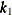and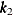) and the value 5 is ignored.

spline(x1 x2/knots=list(1 2 3 4 5))

Table 42.6: Knot Values for a Bivariate Spline with a Supplied List

 x1 x21 23 4

EQUAL(n)

specifies the number of equally spaced interior knots for every variable in a spline term. Two boundary knots are automatically added to the knot list for each variable such that the total number of knots is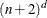, where d is the number of variables in the spline term. For a multivariate spline term, knot values for each variable are determined independently from the corresponding boundary values. For example, if the boundary points for x1 are 1 and 5 and the boundary points for x2 are 2 and 6, then the following specification of a spline term produces nine actual knots (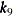), which consist of two boundary knots and one interior knot for each variable.

spline(x1 x2/knots=equal(1))

Table 42.7: Knot Values for a Bivariate Spline with One Interior Knot

 x1 x21 21 4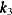1 6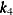3 2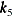3 4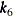3 6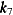5 2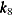5 45 6

M=number

specifies the order of the derivative in the penalty term. The number must be a positive integer. The default is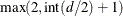, where d is the number of variables in the spline term.

MAXDF=number

specifies the maximum number of degrees of freedom. When a thin-plate regression spline is formed, the specified number is used for constructing a low-rank penalty matrix to approximate the penalty matrix via the truncated eigendecomposition. The number must be greater than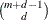, where m is the derivative order that is specified in the M= option. The default is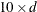. For more information, see the section Thin-Plate Regression Splines.

MAXKNOTS=number

specifies the maximum number of knots if data points are used to form knots. If KNOTS=LIST(list-of-values) is not specified, PROC GAMPL forms knots from unique data points. If the number of unique data points is greater than number, a subset of size number is formed by random sampling from all unique data points. The number cannot exceed the largest integer that can be stored on the given machine. By default, MAXKNOTS=2000.

MAXSMOOTH=number

specifies the upper bound for the smoothing parameter. The default is the largest double-precision value.

MINSMOOTH=number

specifies the lower bound for the smoothing parameter. By default, MINSMOOTH=0.

SMOOTH=number

specifies a fixed smoothing parameter. When you specify this option, no smoothing parameter selection is performed on the spline term.

Model Options

ALLOBS

requests that all nonmissing values of the variables be specified in a spline term for constructing the spline basis functions, regardless of whether other model variables are missing.

CRITERION=criterion

specifies the model evaluation criterion for selecting smoothing parameters for spline-effects. You can specify the following values:

GACV<(FACTOR=number | GAMMA=number)>

uses the generalized approximate cross validation (GACV) criterion to evaluate models.

GCV<(FACTOR=number | GAMMA=number)>

uses the generalized cross validation (GCV) criterion to evaluate models.

UBRE<(FACTOR=number | GAMMA=number)>

uses the unbiased risk estimator (UBRE) criterion to evaluate models.

The default criterion depends on the value of the DISTRIBUTION= option. For distributions that involve dispersion parameters, GCV is the default. For distributions without dispersion parameters, UBRE is the default. For all three criteria, you can optionally use the FACTOR= option to specify an extra tuning parameter in order to penalize more for model roughness. The value of number must be greater than or equal to 1. For more information about the model evaluation criteria, see Model Evaluation Criteria.

DISPERSION=number

specifies a fixed dispersion parameter for distributions that have a dispersion parameter. The dispersion parameter that is used in all computations is fixed at number; it is not estimated.

DISTRIBUTION=keyword

specifies the response distribution for the model. The keywords and their associated distributions are shown in Table 42.8.

Table 42.8: Built-In Distribution Functions

Distribution

DISTRIBUTION=

Function

BINARY

Binary

BINOMIAL

Binary or binomial

GAMMA

Gamma

INVERSEGAUSSIAN | IG

Inverse Gaussian

NEGATIVEBINOMIAL | NB

Negative binomial

NORMAL | GAUSSIAN

Normal

POISSON

Poisson

If you do not specify a link function in the LINK= option, a default link function is used. The default link function for each distribution is shown in Table 42.9. You can use any link function shown in Table 42.10 by specifying the LINK= option. Other commonly used link functions for each distribution are shown in Table 42.9.

FDHESSIAN

requests that the second-order derivatives (Hessian) be computed using finite-difference approximations based on evaluation of the first-order derivatives (gradient). This option might be useful if the analytical Hessian takes a long time to compute.

INITIALPHI=number

specifies a starting value for iterative maximum likelihood estimation of the dispersion parameter for distributions that have a dispersion parameter.

specifies the link function for the model. The keywords and the associated link functions are shown in Table 42.10. Default and commonly used link functions for the available distributions are shown in Table 42.9.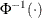denotes the quantile function of the standard normal distribution.

MAXPHI=number

specifies an upper bound for maximum likelihood estimation of the dispersion parameter for distributions that have a dispersion parameter.

METHOD=OUTER | PERFORMANCE

specifies the algorithm for selecting smoothing parameters for spline-effects. You can specify the following values:

OUTER

specifies the outer iteration method for selecting smoothing parameters. For more information about the method, see the section Outer Iteration.

Note: The outer iteration method is experimental in this release.

PERFORMANCE

specifies the performance iteration method for selecting smoothing parameters. For more information about the method, see the section Performance Iteration.

By default, METHOD=PERFORMANCE.

MINPHI=number

specifies a lower bound for maximum likelihood estimation of the dispersion parameter for distributions that have a dispersion parameter.

NORMALIZE

requests normalized spline basis functions for model fitting. After the regression spline basis functions are computed, each column is standardized to have a unit standard error. The corresponding penalty matrix is also scaled accordingly. This option might be helpful when you have badly scaled data.

OFFSET=variable

specifies a variable to be used as an offset to the linear predictor. An offset plays the role of an effect whose coefficient is known to be 1. The offset variable cannot appear in the CLASS statement or elsewhere in the MODEL statement. Observations that have missing values for the offset variable are excluded from the analysis.

RIDGE=number

allows a ridge parameter such that a diagonal matrix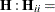number is added to the optimization problem with respect to regression parameters: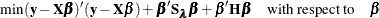By default, RIDGE=0. Specifying a small ridge parameter might be helpful if the model matrix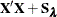is close to singular.

SCALE=DEVIANCE | MLE | PEARSON

specifies the method for estimating the scale and dispersion parameters. You can specify the following values:

DEVIANCE

estimates the dispersion parameter by using the deviance statistic.

MLE

computes the dispersion parameter by maximizing the likelihood or penalized likelihood.

PEARSON

estimates the dispersion parameter by using Pearson’s statistic.

By default, SCALE=MLE.